The division with the pascaline

The division example of the manuscript "Usage de la machine" takes again the same numbers as for the multiplication. We want divide 23688 by 56. We firstly initialize the machine and set it in the substraction position (the sliding cover is shifted toward the operator). The dividend is then set in the display windows (the same manner we do for the substraction). The divisor is written on a strip of paper leaving between the digits as much space as it exists between the display windows. The strip is positioned at the left side.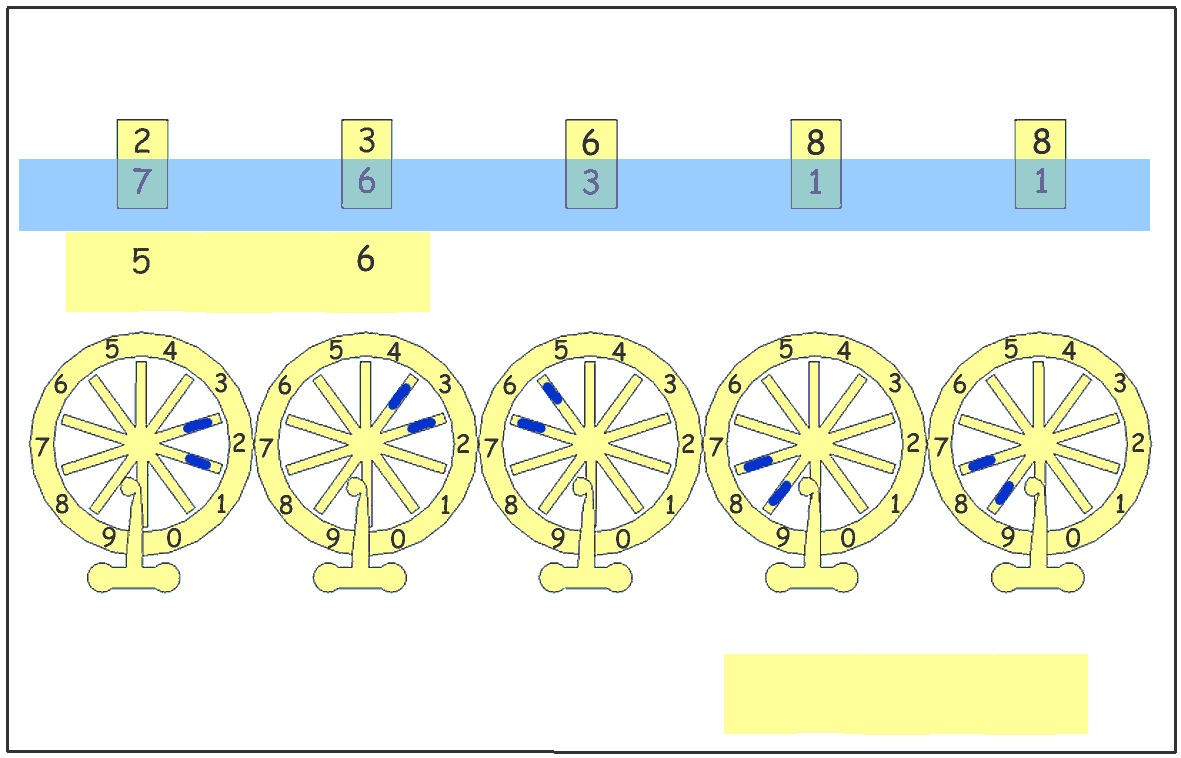Before making the division, the author of the manuscript explain how to manage this operation.

"[...] Because it can happen that a too large digit is obtained for the quotient if the first digit of the divisor is exactly entered, I take it always greater of one unit of his value [...] I then use this quotient to multiply the divisor, after entering in the machine the digits this multiplication gives me I see in the display windows the remainder of the division. If the remainder is greater than the divisor, this means that the quotient must be larger by 1. In this case I add 1 at the bottom of the written quotient."

Suppose I want divide 437 by 23, I consider how many times 3 (2 + 1) is in 4. The answer is 1. When I substract once 23 from 43 the remainder is equal to 20 who is lesser than 23. Then the first digit of the quotient who is 1 is exact.

Instead of 437, suppose I want to divide 475 by 23. As above, I consider how many times 3 (2 + 1) is in 4. The answer is 1. When I substract once 23 from 47 the remainder is equal to 24 who is greater than 23. Then it is possible to substract one more time. Then the first digit of the quotient is not 1 but is equal to 2.

Let us come back to the division of 23688 by 56 :

" [...] I then write 56 on the strip and I put it under the two first digits at the left of the dividend who are 23. The number 56 exceeds the number 23, then I can not work with these two numbers. I am obliged to shift right the strip of one position in order to align the digits 5 and 6 under the second and the third digits of the dividend [...] Then I am able to look for how many times 56 is in 236."

a) Finding the first digit of the quotient :

With the explained method, we consider how many times the first digit of the divisor enlarged by 1 is in 23. The multiplication table says 3. The first digit of the quotient is 3 (at this time). One substracts 3 x 56 from 236.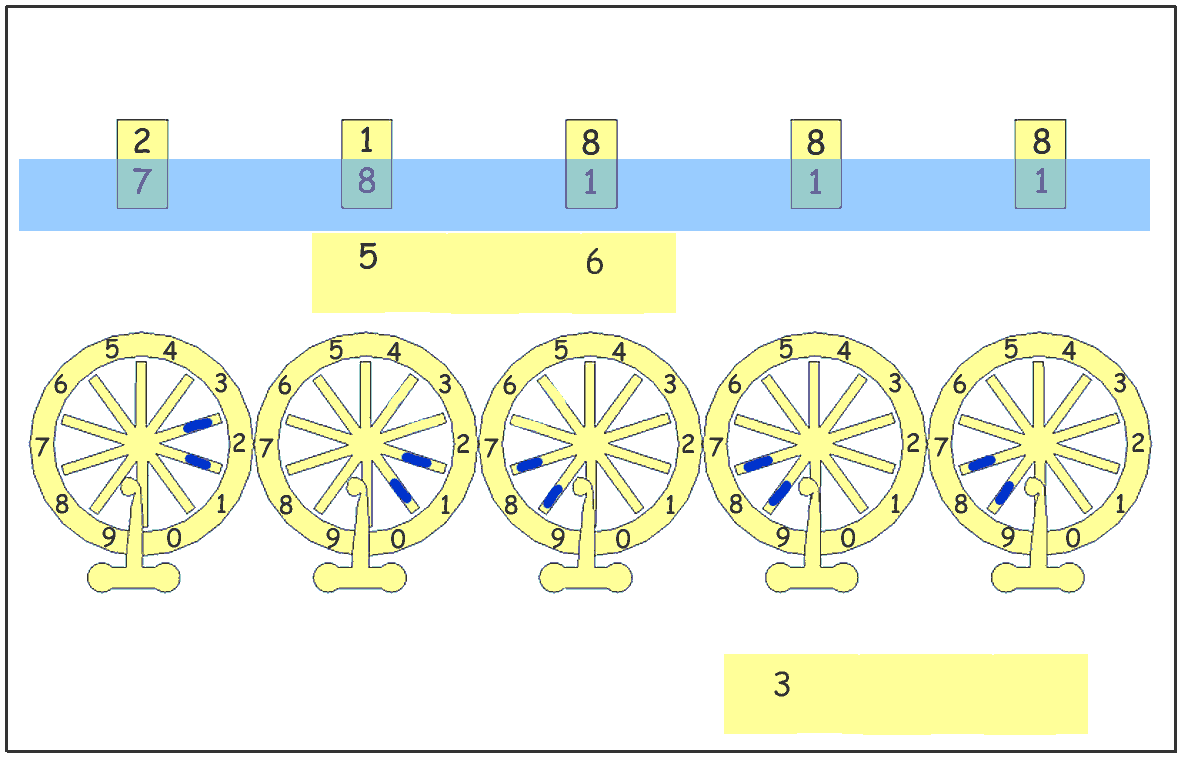3 x 6 = 18 => 18 is entered on the wheels with the digit "8" below the digit "6" of 56.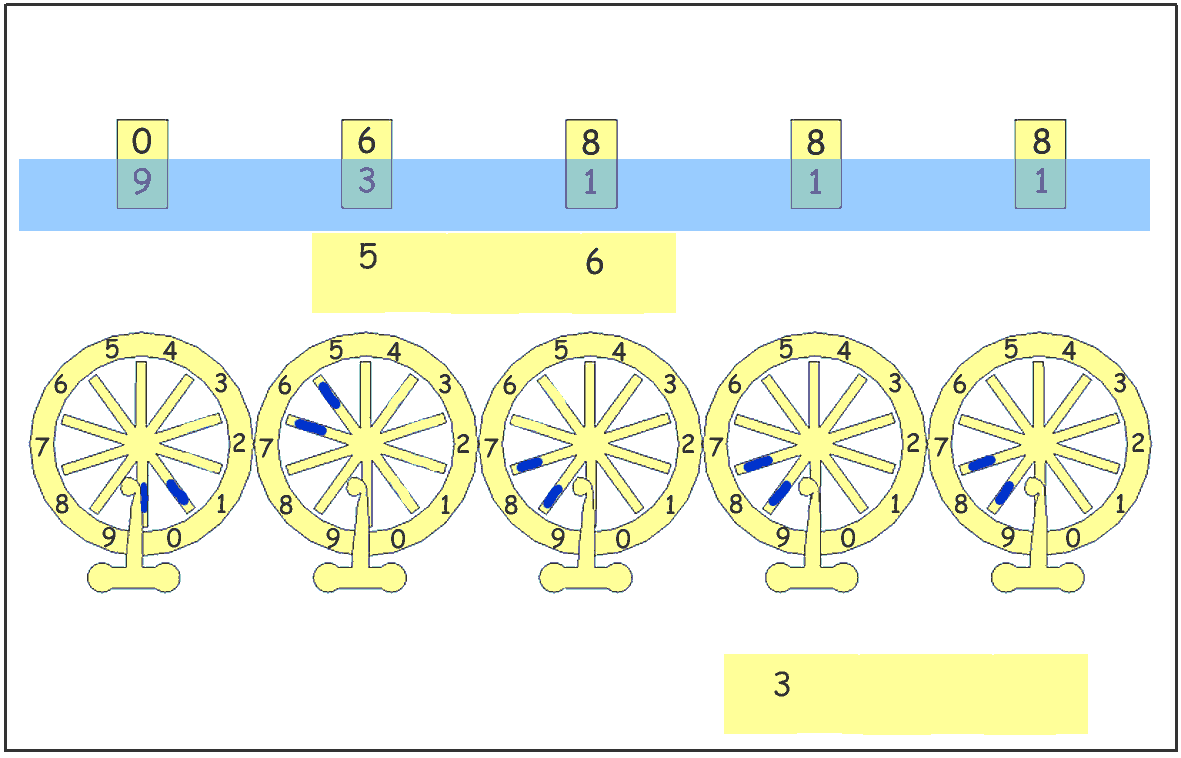3 x 5 = 15 => 15 is entered on the wheels with the digit "5" below the digit "5" of 56.

The result 6888 comes in the display windows, the number 56 on the strip of paper being under 68. As we can see, it is possible to substract 56 one more time. One concludes that the first digit of the quotient is not 3 as we found it but is equal to 4.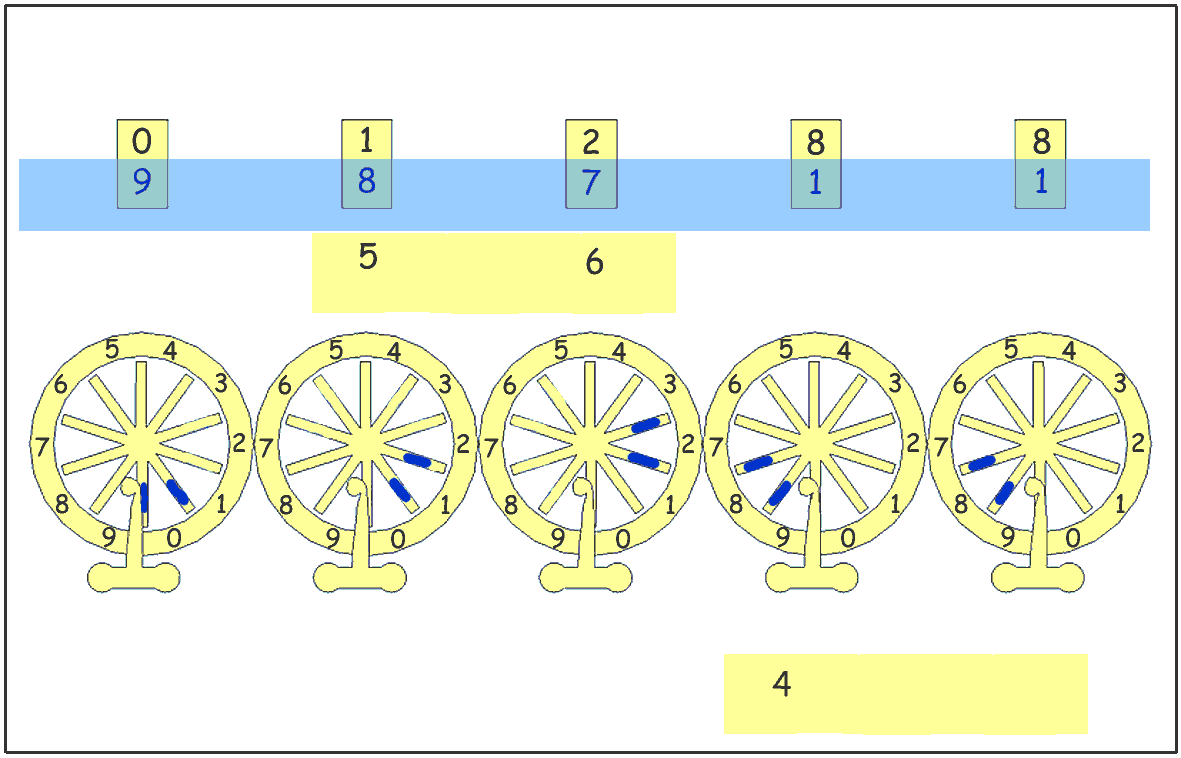When we substract 56 one more time (we just have to enter 56 on the wheels) 1288 comes in the display windows. The number 56 of the strip of paper is under the number 12.

 The manuscript "Usage de la machine" ask the operator to provide itself with a multiplication table (called “booklet”, schematized opposite). It specifies that it is easy to memorize this table. The films which follow suppose that the operator memorized the multiplication table.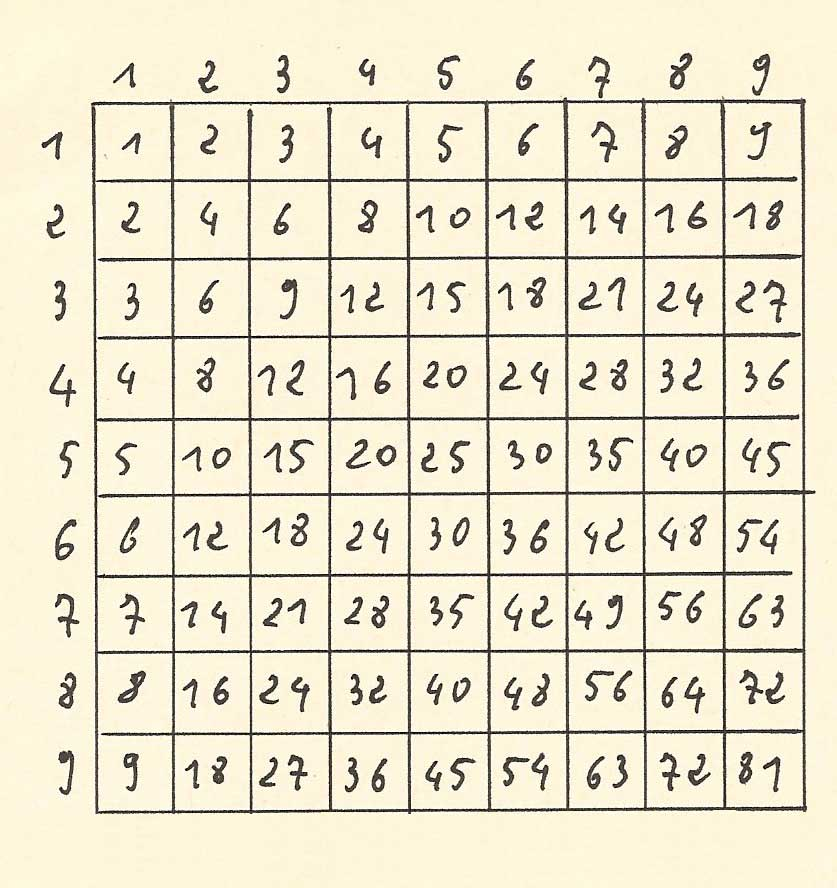The following movie shows how to calculate the first figure of the quotient.b) Finding the second digit of the quotient :

The paper strip is shifted one position to the right. 56 is now under the digits 28 of the number 128. We do as above.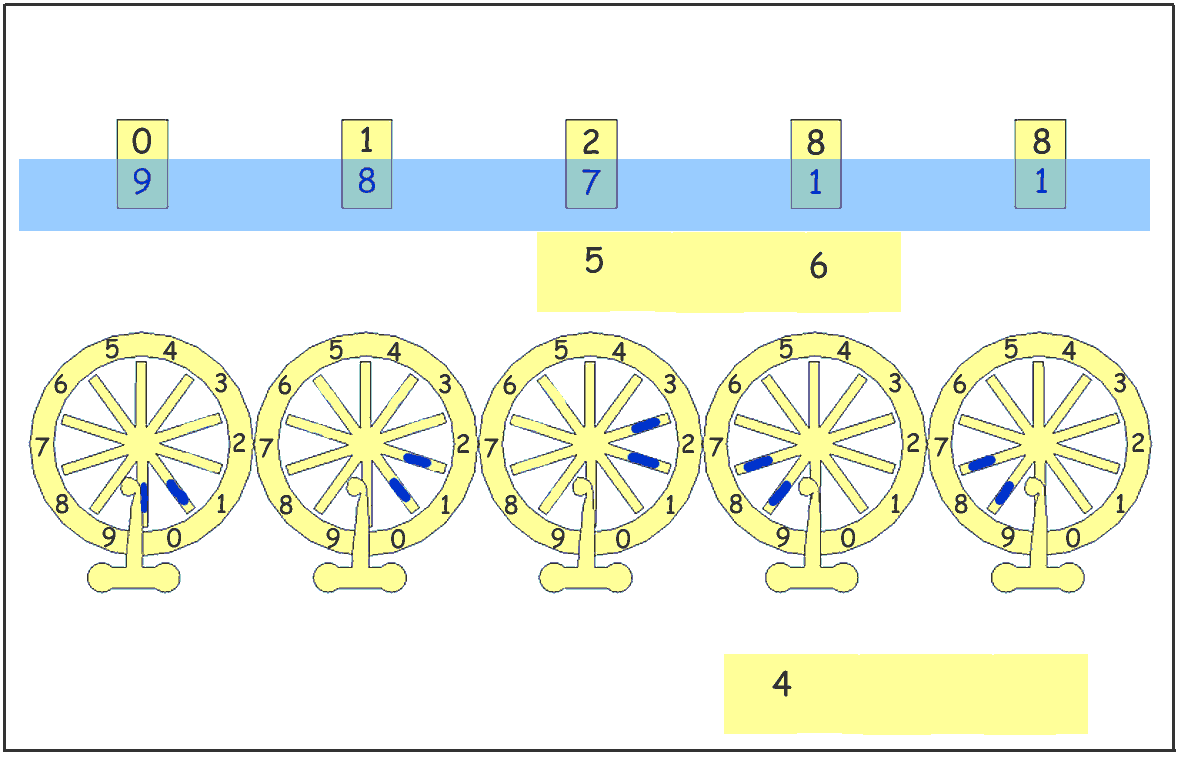How many times 6 (5 + 1) is in the number 12. The multiplication table says 2. Then the second digit of the quotient is 2 (for the moment). We substract 2 x 56 from 128.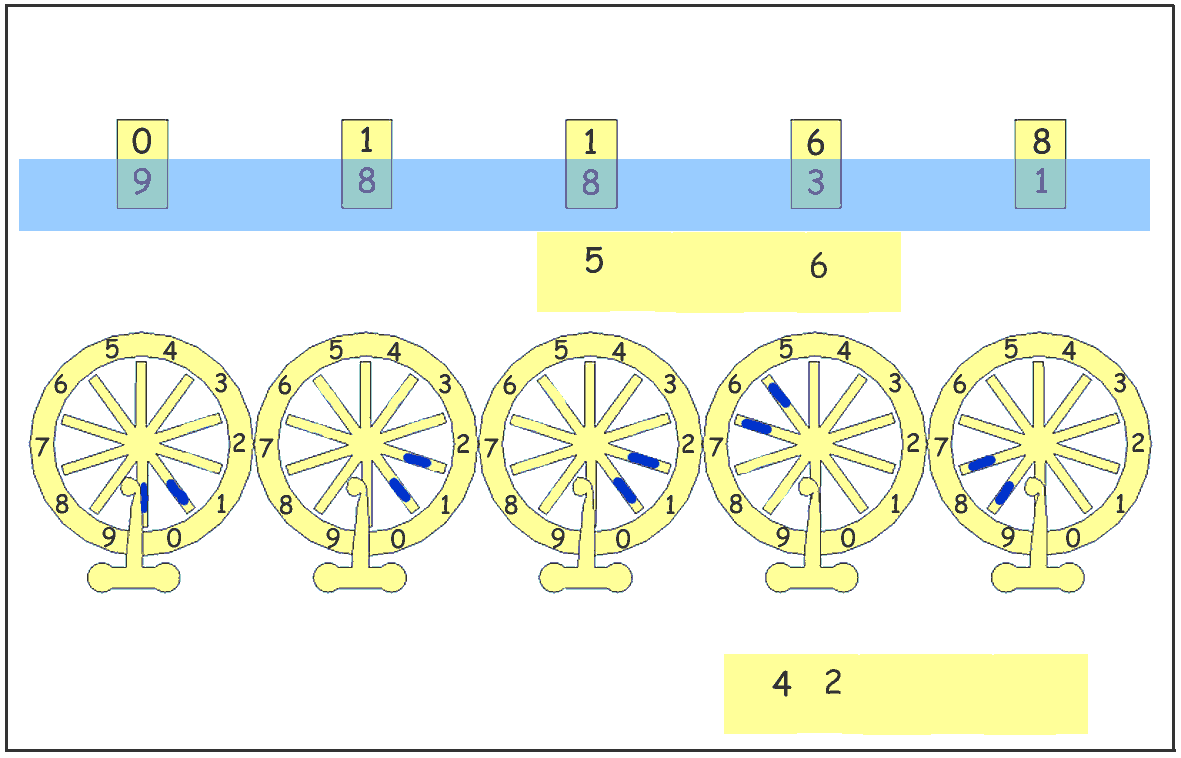2x 6 = 12 => 12 is entered on the wheels with the digit "2" below the digit "6" of 56.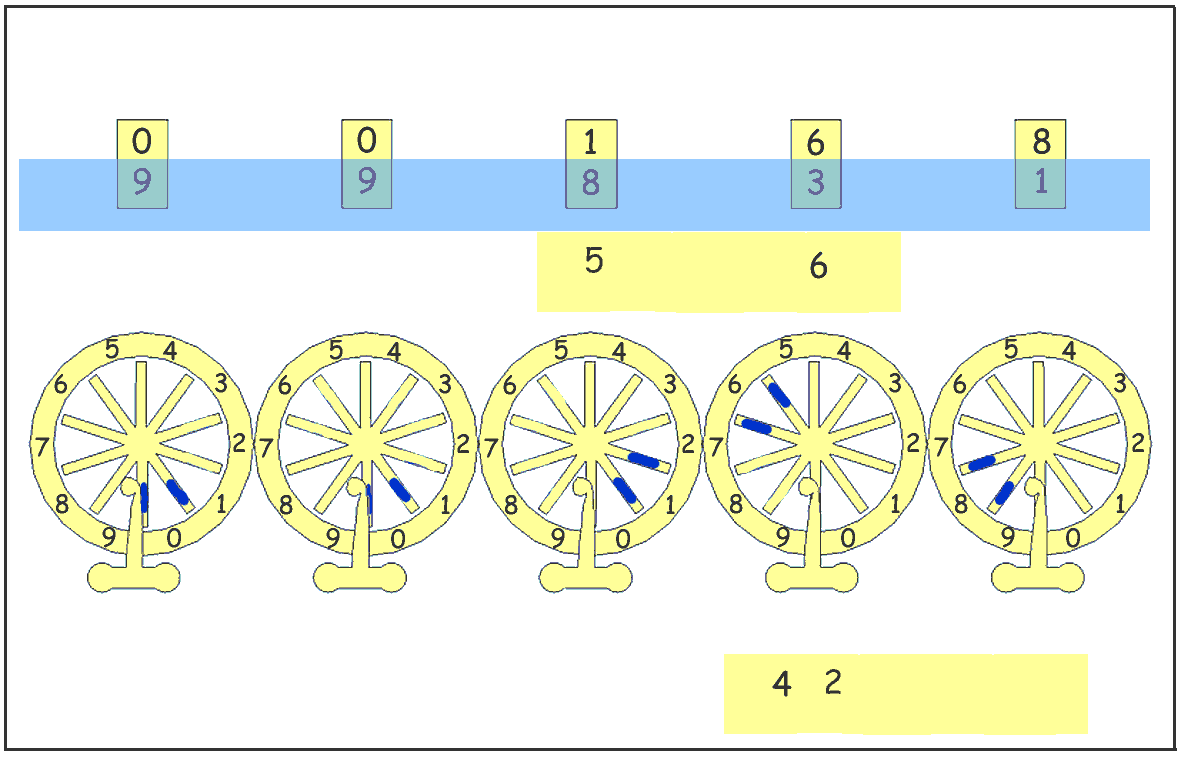2 x 5 = 10 => 10 is entered on the wheels with the digit "0" below the digit "5" of 56.

The result 168 is displayed in the windows, the number 56 of the paper strip being under the number 16. Now 16 is lower to 56, consequently the second digit of the quotient is correct.

The movie shows how to calculate the second figure of the quotient.c) Finding the third digit of the quotient :

The paper strip is shifted one position to the right. 56 is now under the digits 68 of the number 168.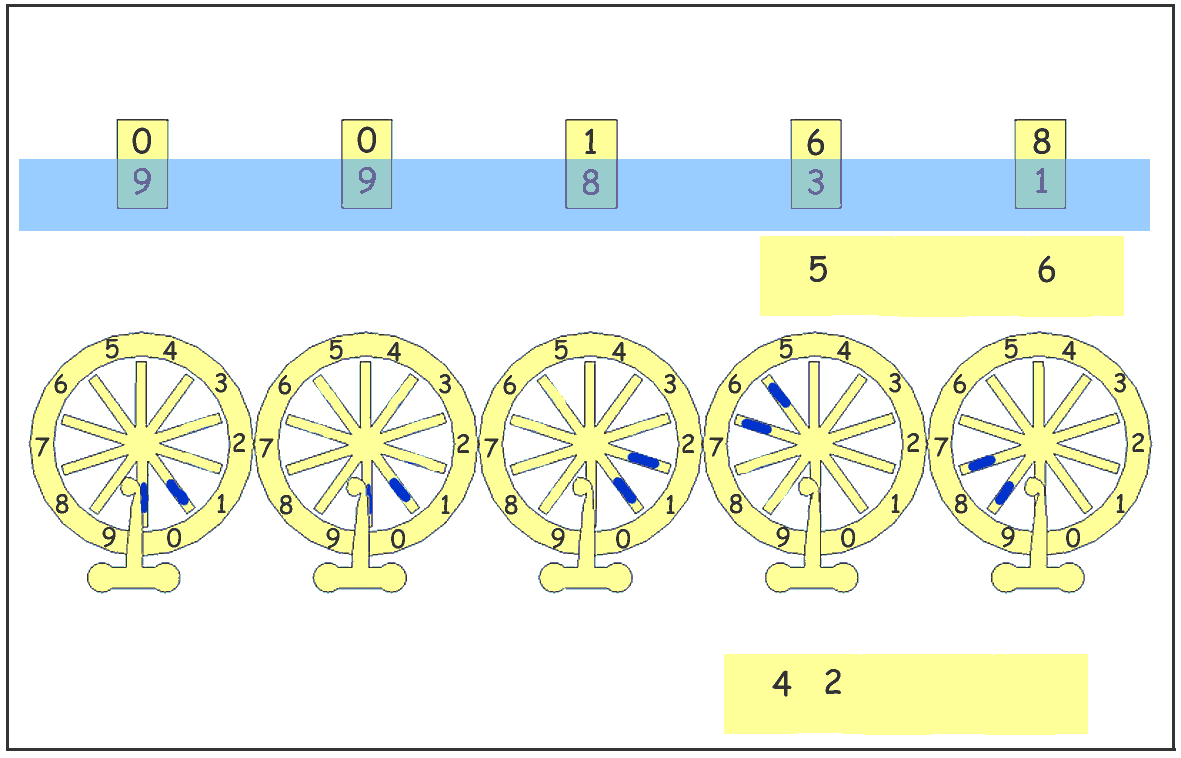How many times 6 (5 + 1) is in the number 16. The multiplication table says 2. Then the third digit of the quotient is 2 (for the moment). We substract 2 x 56 from 168.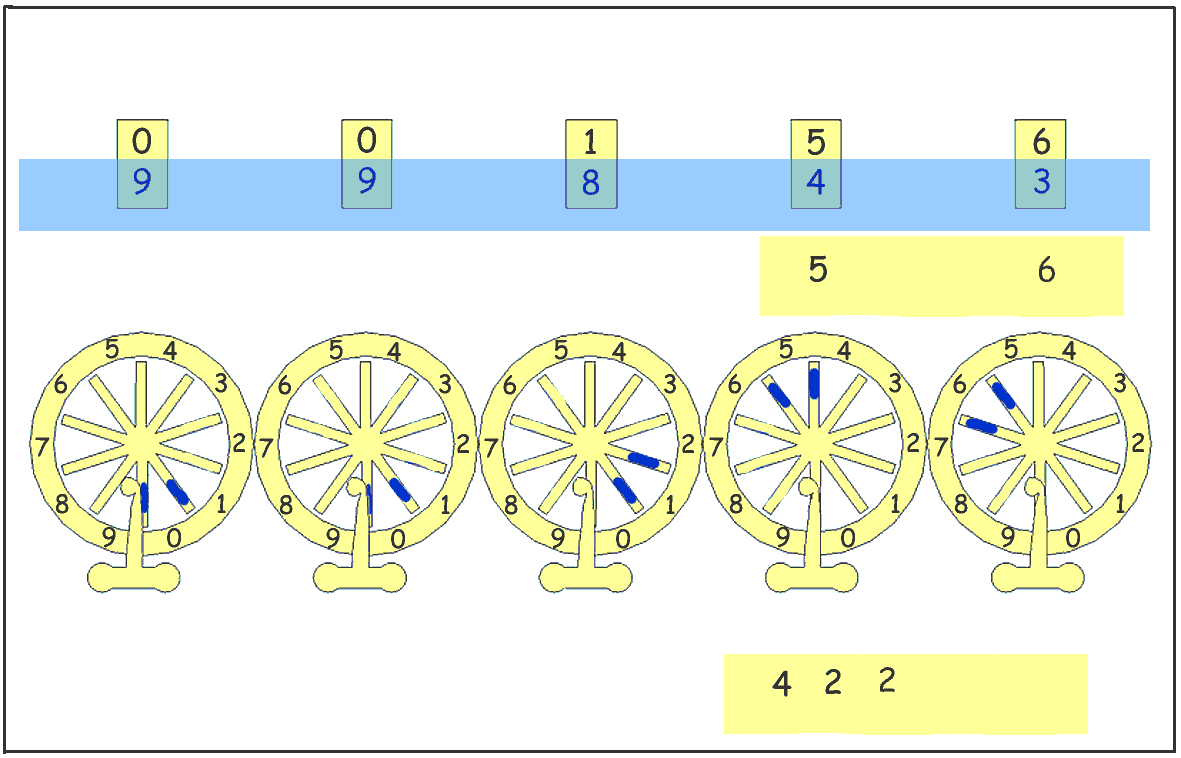2x 6 = 12 => 12 is entered on the wheels with the digit "2" below the digit "6" of 56.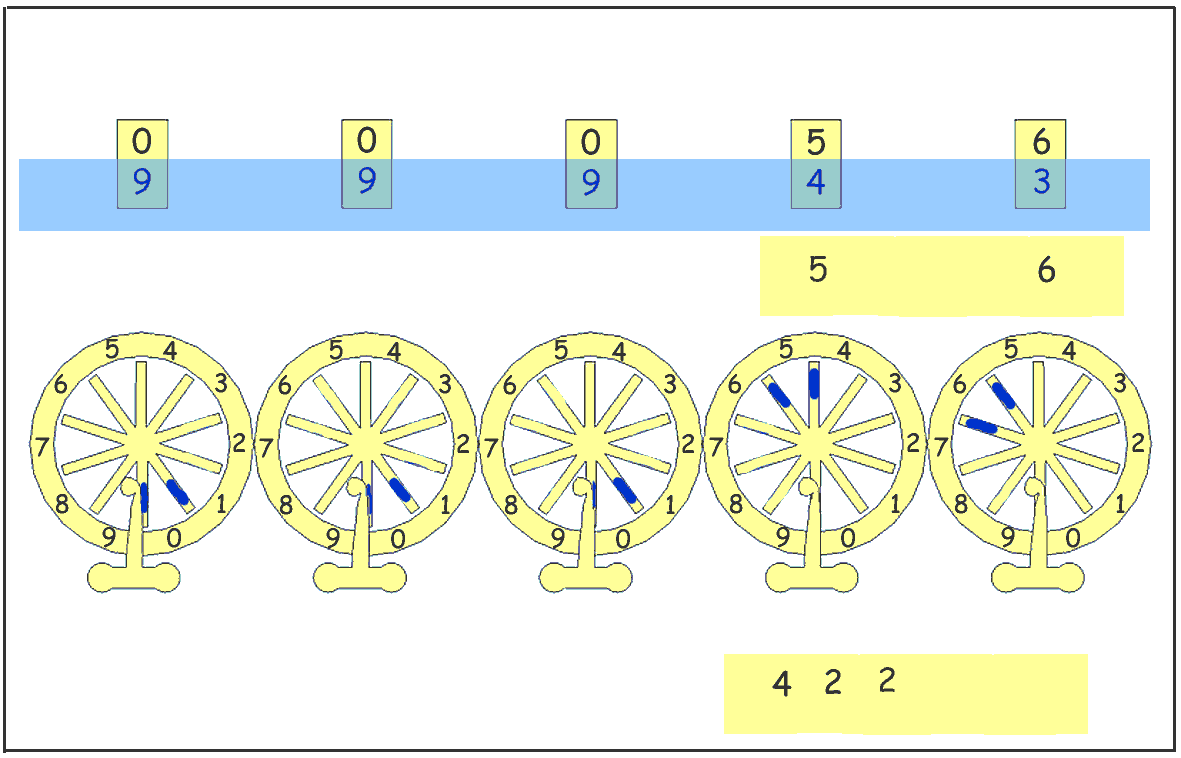2 x 5 = 10 => 10 is entered on the wheels with the digit "0" below the digit "5" of 56.

The display windows show 56. This means we can substract 56 one more time. The quotient must be increased by 1, it becomes 3 and the last substraction gives a null result. The division has no remainder.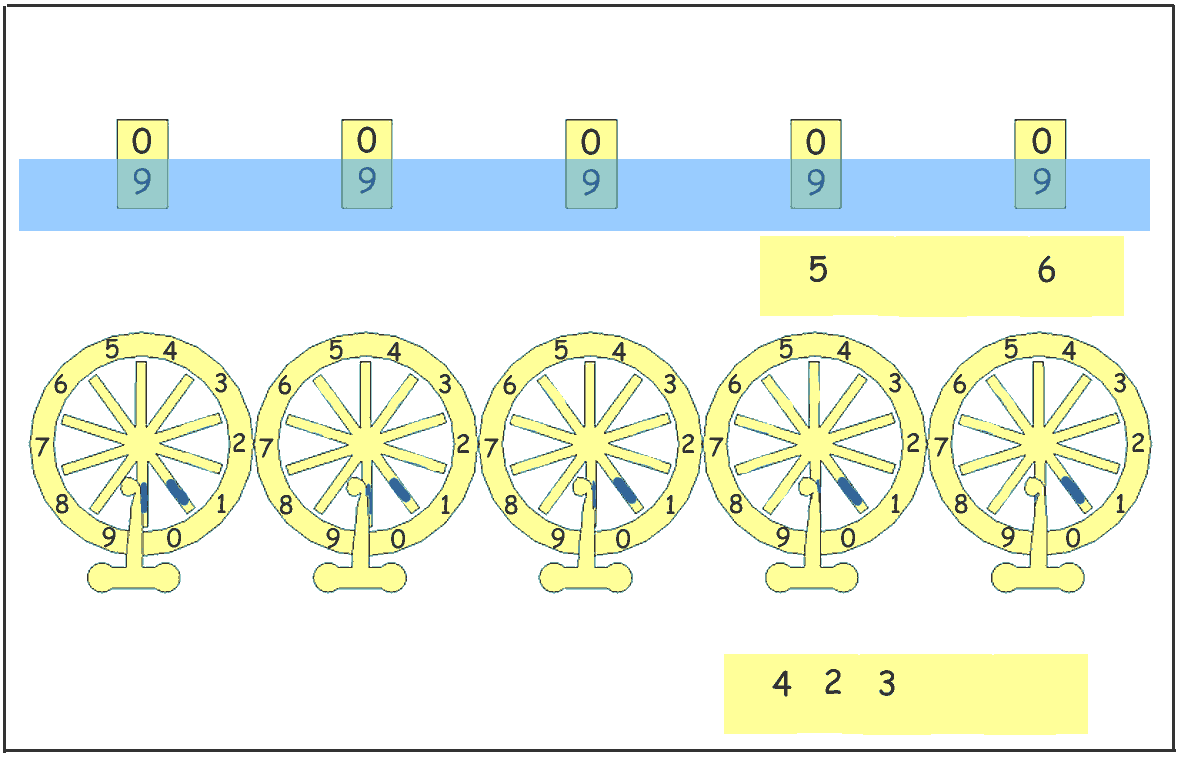We finally obtain 23688 / 56 = 423.

The film below illustrates the stages leading to the last figure of the result.The manuscript gives a detailed explanation to perform the division. Nevertheless, the basic operative technic is simple and repetitive. The conclusion is that if the division was not so simple than the addition, she was feasable with the pascaline (with the described method of the 18th century manuscript).